# 数据结构和算法7-搜索

## 1.顺序表

• 顺序查找
• 二分查找

### 1-1.顺序查找

``````public class LinearSeqSearch {
public static void main(String[] args) {
// 无序的
int[] data = {1, 4, 3, 6, 5, 2, 7};
// int checkNum = 7;
int checkNum = 100;
int index = find(checkNum, data);
if (index < 0) {
System.out.println("没有找到："+ checkNum);
}else {
System.out.println("坐标："+ index);
}

}

private static int find(int check, int[] data) {
for (int index = 0; index < data.length; index++) {
if (check == data[index]) {
return index;
}
}
return -1;
}
}
``````

### 1-2.二分查找

• 非递归：空间复杂度S(n)=O(1);
• 递归：调了logn次方法，所以空间复杂度是O(logn)

``````blic class LinearBinarySearch {
public static void main(String[] args) {
// 折半查找的数据要是有序的
int[] data = {1, 2, 3, 4, 5, 6, 7, 10};
// int checkNum = 6;
int checkNum = 9;

// 非递归
int index = findByBinaryNoRec(checkNum, data);

if (index < 0) {
System.out.println("没有找到：" + checkNum);
} else {
System.out.println("坐标：" + index);
}
}

private static int findByBinaryNoRec(int check, int[] data) {
int minIndex = 0;
int maxIndex = data.length - 1;

while (maxIndex > minIndex) {
int midIndex = (maxIndex + minIndex) / 2;
if (data[midIndex] == check) {
return midIndex;
} else if (data[midIndex] > check) {
// 注意着要-1，因为中间的值的已经判断过了
maxIndex = midIndex - 1;
} else {
// 注意着要+1，因为中间的值的已经判断过了
minIndex = midIndex + 1;
}
}
return -1;
}
}
``````

``````public class LinearBinarySearch {
public static void main(String[] args) {
// 折半查找的数据要是有序的
int[] data = {1, 2, 3, 4, 5, 6, 7, 10};
// int checkNum = 6;
int checkNum = 9;

// 递归
int index = findByBinaryRec(checkNum, data);

if (index < 0) {
System.out.println("没有找到：" + checkNum);
} else {
System.out.println("坐标：" + index);
}
}

private static int findByBinaryRec(int check, int[] data) {
return findByBinaryRec(0, data.length - 1, check, data);
}

private static int findByBinaryRec(int minIndex, int maxIndex, int check, int[] data) {

if (minIndex >= maxIndex) {
return -1;
}

int midIndex = (minIndex + maxIndex) / 2;
if (data[midIndex] == check) {
return midIndex;
} else if (data[midIndex] > check) {
return findByBinaryRec(minIndex, midIndex - 1, check, data);
} else {
return findByBinaryRec(midIndex + 1, maxIndex, check, data);
}
}
}
``````

### 1-3.插值法

`mid=(high+low)/2 = low + (high-low)/2` 这是等价的。但是这个`1/2`是固定的，不好。可以进化下，根据数据的特点，动态进行调幅。

``````public class LinearInsertSearch {
public static void main(String[] args) {
// 折半查找的数据要是有序的
int[] data = {1, 2, 3, 4, 5, 6, 7, 10};
int checkNum = 6;
// int checkNum = 9;

// 非递归
int index = findByBinaryNoRec(checkNum, data);

// 递归
// int index = findByBinaryRec(checkNum, data);

if (index < 0) {
System.out.println("没有找到：" + checkNum);
} else {
System.out.println("坐标：" + index);
}

}

private static int findByBinaryRec(int check, int[] data) {

return findByBinaryRec(0, data.length - 1, check, data);
}

private static int findByBinaryRec(int minIndex, int maxIndex, int check, int[] data) {

if (minIndex >= maxIndex) {
return -1;
}

// int midIndex = (minIndex + maxIndex) / 2;
int midIndex = minIndex + (check - data[minIndex]) / (data[maxIndex] - data[minIndex]) * (maxIndex - minIndex);
if (data[midIndex] == check) {
return midIndex;
} else if (data[midIndex] > check) {
return findByBinaryRec(minIndex, midIndex - 1, check, data);
} else {
return findByBinaryRec(midIndex + 1, maxIndex, check, data);
}
}

private static int findByBinaryNoRec(int check, int[] data) {
int minIndex = 0;
int maxIndex = data.length - 1;

while (maxIndex > minIndex) {
// int midIndex = (maxIndex + minIndex) / 2;
int midIndex = minIndex + (check - data[minIndex]) / (data[maxIndex] - data[minIndex]) * (maxIndex - minIndex);
if (data[midIndex] == check) {
return midIndex;
} else if (data[midIndex] > check) {
// 注意着要-1，因为中间的值的已经判断过了
maxIndex = midIndex - 1;
} else {
// 注意着要+1，因为中间的值的已经判断过了
minIndex = midIndex + 1;
}
}
return -1;
}
}
``````

## 2.查找树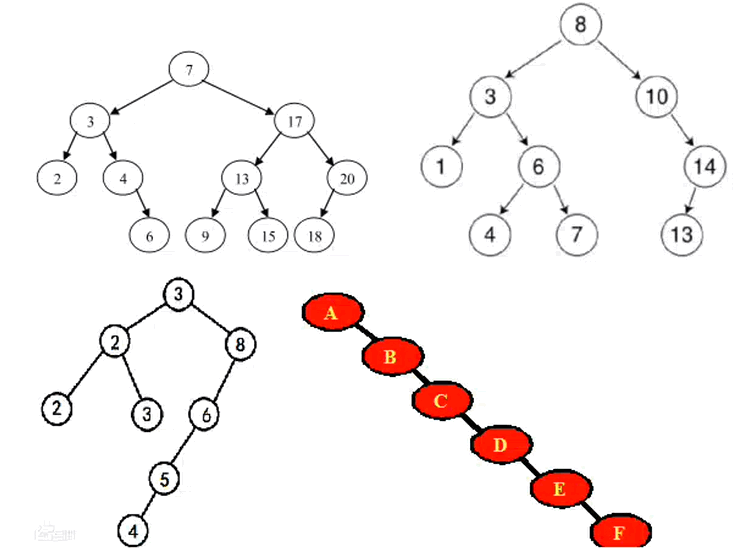### 2-1.平衡二叉树

• 左右两个子树的高度差（平衡因子）的绝对值不超过1, 子树的子树也要保证这个关系。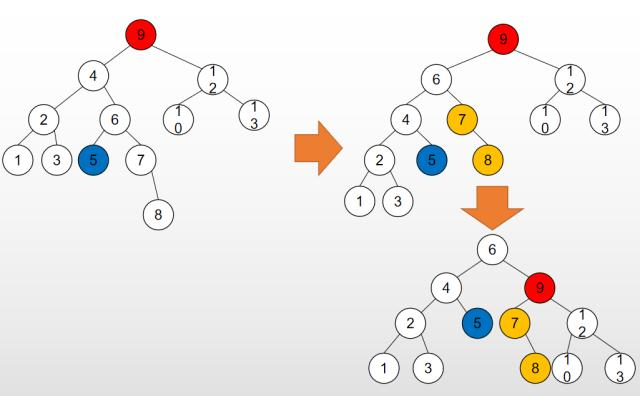### 2-2.红黑树

Red-Black Tree 简称 R-B Tree。也是平衡二叉树，每个节点上都存储位颜色，红或黑。

• 每个节点不是黑就是红
• 根是黑的
• 叶子是NIl是黑的
• 一个节点是红的，那么子节点必是黑的
• 从一个节点到子孙节点所有路径包含相同数目的黑节点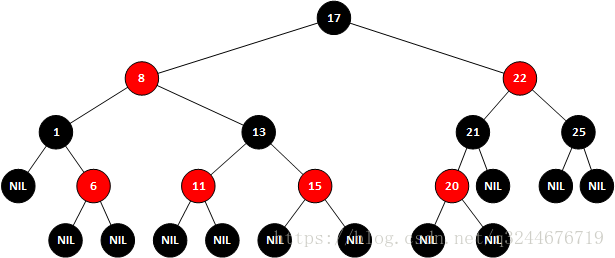java中的TreeSet和TreeMap都是使用红黑树实现的
linux的内存管理也是

### 2-3.B树

B树，相对于二叉树，叉树多了，可以降低树的升读，提交查询效率

B树是因为文件系统而发展起来的，大量数据放放磁盘中，数据量太大，不能一次加载进去，需要需要索引，来提高访问速度，减少时间。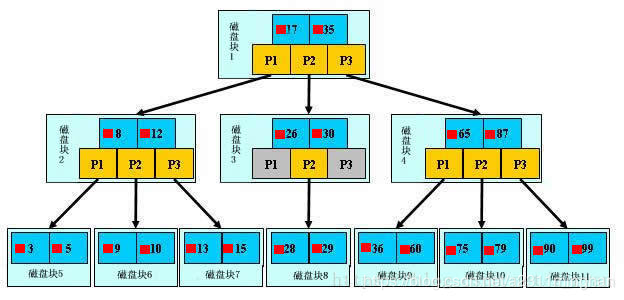### 2-4.B+树

Ｂ＋树是Ｂ树的变种，有着比Ｂ树更高的查询效率。

• 每个元素不保存数据，只用来索引，所有数据都保存在叶子节点
• 所有的叶子结点中包含了全部元素的信息，及指向含这些元素记录的指针，且叶子结点本身依关键字的大小，自小而大顺序链接。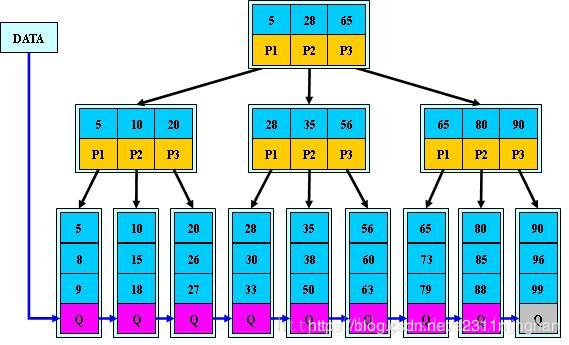B+树通常有两个指针，一个指向根结点，另一个指向关键字最小的叶子结点。因些，对于B+树进行查找两种运算：一种是从最小关键字起顺序查找，另一种是从根结点开始，进行随机查找。

### 2-5.B*树

B*是B+树的变体，在B+树的非根和非叶子结点再增加指向兄弟的指针；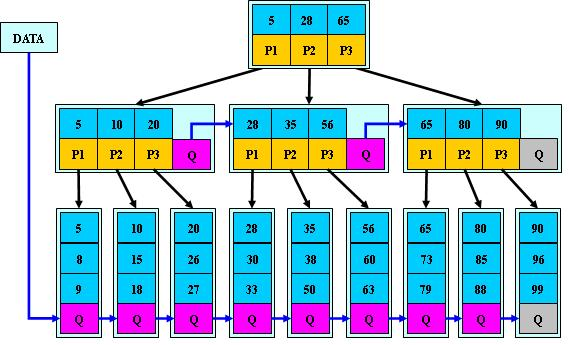## 3.hash表

### 3-1.hash表结构

hashtable也叫散列表。查询、插入速度快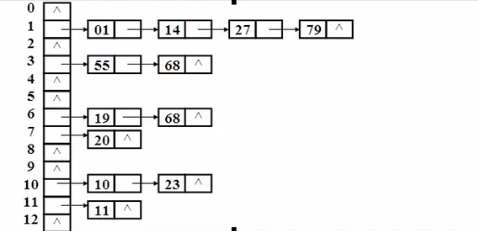### 3-2.哈希码

• int取自身
• double 源码，挺复杂的还有位运算和取或
• String
• 具体的类，但是属性是基本类型，终归是可以得到一个整数的。(idea、eclipse工具自动生成)

### 3-3.hash冲突

• 直接定址法
• 平方取中发
• 折叠法
• 除留取余法（y=x%11）

hash函数解决冲突的方法有以下几个常用的方法

• 开放定制法
• 链地址法
• 公共溢出区法

• 再散列法

## 4.进入一步深造

TreeSet与TreeMap

• java中的TreeSet和TreeMap的底层就是使用了红黑树。
• 添加节点的过程很通过旋转等方法，使得每次添加数据后得到的都是平衡树；
• TreeSet给你创建了一个TreeMap，放到了Map的key里面，而map的value都是一个新的Object对象

HashSet和HashMap

• HashSet的底层使用HashMap，使用了一个是哈希表。
• JDK1.7的HashMap是一个table数组+链表实现的存储结构
• JDK.18的HashMap当链表的存储数据个数大于等于8的时候，不再用链表存储，而是采用的红黑树存储。